Search
Document Center

# Financial algorithms

Last Updated: Aug 17, 2018

## Binning

Binning with equal frequency or equal width.

#### PAI command

PAI -name binning    -project algo_public     -DinputTableName=input     -DoutputTableName=output

#### Parameter description

Parameter Description Option Default value
inputTableName (Required) Name of the input table table name NA
outputTableName (Required) Name of the output table table name NA
selectedColNames (Optional) Binning columns in the input table column name All columns except the label column, or all columns when no label exists
labelColumn (Optional) Label column column name No label
validTableName (Optional) Validation table name entered when binningMethod is auto. Required in auto mode. table name Null
validTablePartitions (Optional) Partitions selected for the validation table partition name Entire table selected
inputTablePartitions (Optional) Names of the selected partitions in the input table partition name All partitions are selected by default.
inputBinTableName (Optional) Input binning table table name No binning table
selectedBinColNames (Optional) Columns selected in the binning table column name Null
positiveLabel (Optional) Type of output positive samples NA 1
nDivide (Optional) Number of bins positive integer 10
colsNDivide (Optional) Number of bins in custom columns, for example, col0:3 and col2:5 The columns that are selected in colsNDivide but are not included in selectedColNames are also calculated. For example, the value is calculated based on col0:3, col1:10, and col2:5 if selectedColNames is col0 and col1, colsNDivide is col0:3 and col2:5, and nDivide is 10. positive integer Null
isLeftOpen (Optional) Interval open mode: left open and right closed, or left closed and right open true/false true
stringThreshold (Optional) Threshold of discrete values in other bins NA No other bins by default
colsStringThreshold (Optional) Threshold of custom columns, the same as colsNDivide NA Null
binningMethod (Optional) Binning method. quantile: equal frequency binning; bucket: equal width binning; auto: monotonous binning automatically selected in quantile mode quantile, bucket, and auto quantile
lifecycle (Optional) Life cycle of the output table positive integer Unspecified by default
coreNum (Optional) Number of cores positive integer Automatically calculated by default
memSizePerCore (Optional) Size of memory positive integer Automatically calculated by default

## Data conversion module

#### Parameter description

Parameter Description
inputFeatureTableName (Required) Name of the input feature table
inputBinTableName (Required) Name of the input binning result table
inputFeatureTablePartitions (Optional) Partitions selected in the input feature table. Entire table selected by default.
outputTableName (Required) Name of the output table
featureColNames (Optional) Names of feature columns selected in the input table. Entire table selected by default.
metaColNames (Optional) Columns without data conversion. The selected columns are output without any change. (No meta column by default, and you can specify label, sample_id, and other columns.)
transformType (Optional) Data conversion method, which is normalize (normalization), dummy (discretization), or woe (WOE conversion). The default value is “dummy”.
itemDelimiter (Optional) Feature delimiter. Default value: comma. Valid only for discretization.
kvDelimiter (Optional) KV delimiter. Default value: colon. Valid only for discretization.
lifecycle (Optional) Output table lifecycle. No lifecycle by default.
coreNum (Optional) Number of CPU cores used (calculated automatically by default)
memSizePerCore (Optional) Memory size used by each CPU core, in MB. Calculated automatically by default.

#### Example

PAI -name data_transform-project algo_public-DinputFeatureTableName=feature_table-DinputBinTableName=bin_table-DoutputTableName=output_table-DmetaColNames=label-DfeatureColNames=feaname1,feaname2

#### Normalization algorithm

Normalization converts variable values into values between 0 and 1 based on input binning information, and sets missing values to 0. The following algorithm is used.

if feature_raw_value == null or feature_raw_value == 0 then    feature_norm_value = 0.0else    bin_index = FindBin(bin_table, feature_raw_value)    bin_width = round(1.0 / bin_count * 1000) / 1000.0    feature_norm_value = 1.0 - (bin_count - bin_index - 1) * bin_width

#### Output format

Normalization and WOE conversion output tables are normal tables.

When variables are converted into dummy variables through discretization, the output table is in KV format and the output variable format is [${feaname}]\_bin\_${bin_id}. For example:

• If the sns variable falls into bin 2, the output variable is [sns]_bin_2.
• If the variable is null, it falls into the null bin and the output variable is [sns]_bin_null.
• If the variable is not null and does not fall into any defined bin, it falls into the else bin and the output variable is [sns]_bin_else.

## Scorecard training

The scorecard is a modeling tool commonly used in credit risk evaluation. Scorecard training discretizes the original variables by binning input and uses a linear model (such as logistic regression and linear regression) to carry out model training. The scorecard supports various features, including feature selection and score conversion. In addition, you can add constraints to variables during model training.

Note: If no binning input is specified, scorecard training is completely equivalent to common logistic regression or linear regression.

#### Feature engineering

The scorecard model differs from ordinary linear models in that it performs feature engineering on the data before the linear model is used for training. The scorecard provides two feature engineering modes, both of which need to discretize the features through binning.

• One method is to generate N dummy variables (N is the number of variable bins) for each variable through One-Hot encoding based on the binning result.
• The other is WOE conversion that replaces the original values of variables with the WOE values of the bins where the variables fall.

Note: When using dummy variables for conversion, you can set constraints between dummy variables of each original variable. For more information, see the subsequent chapters.

#### Score conversion

In scorecard scenarios such as credit scoring, the odds of predicted samples must be transformed into scores by linear transformation, typically as follows:

***log(odds) = \sum(w*x) = a * scaled_score + b***

Specify the linear transformation relationship by using the following parameters:

• scaledValue: Specifies a benchmark for a score.
• odds: Specifies the odds value at a given score benchmark.
• pdo(Point Double Odds): Doubles the odds for multiple scores.

For example, if scaledValue is 800, odds is 50, and pdo is 25, the two points in a straight line are specified.

log(40) = a * 800 + b

log(80) = a * 825 + b

• Calculate a and b, and conduct linear transformation on the scores in the model to obtain the transformed variables.

• The scaling information is specified by the -Dscale parameter in the JSON format, as follows:

{"scaledValue":800,"odds":50,"pdo":25}
• When this parameter is not null, set the values of all the preceding three parameters.

#### Support constraints in the training process

In the scorecard training, you can add constraints to variables. The implementation of constraints depends on the underlying constrained optimization algorithm. Set the constraints in the binning UI. After the setting, the bin generates a constraint in JSON format and automatically transfers it to the training components connected. The scorecard training component supports the following method to add constraints to variables:

• Specify the score of a certain bin to be a fixed value.
• Specify the scores of two bins to a certain proportion.
• Limit the scores between bins, such as sorting bin scores by the WOE values in the bins.

The following is a demonstration of binning component operations: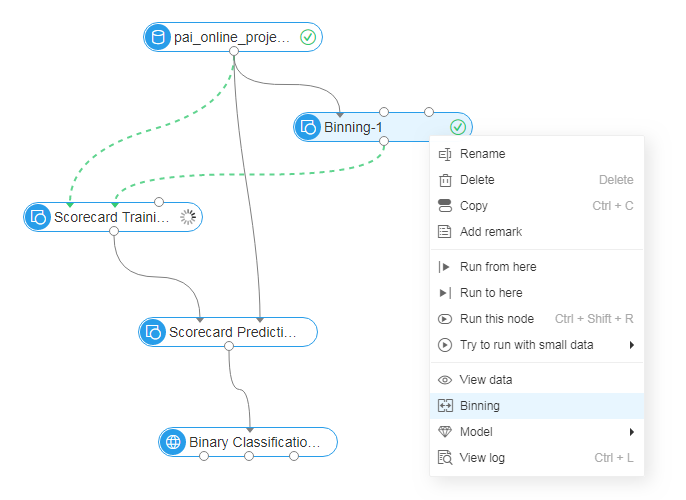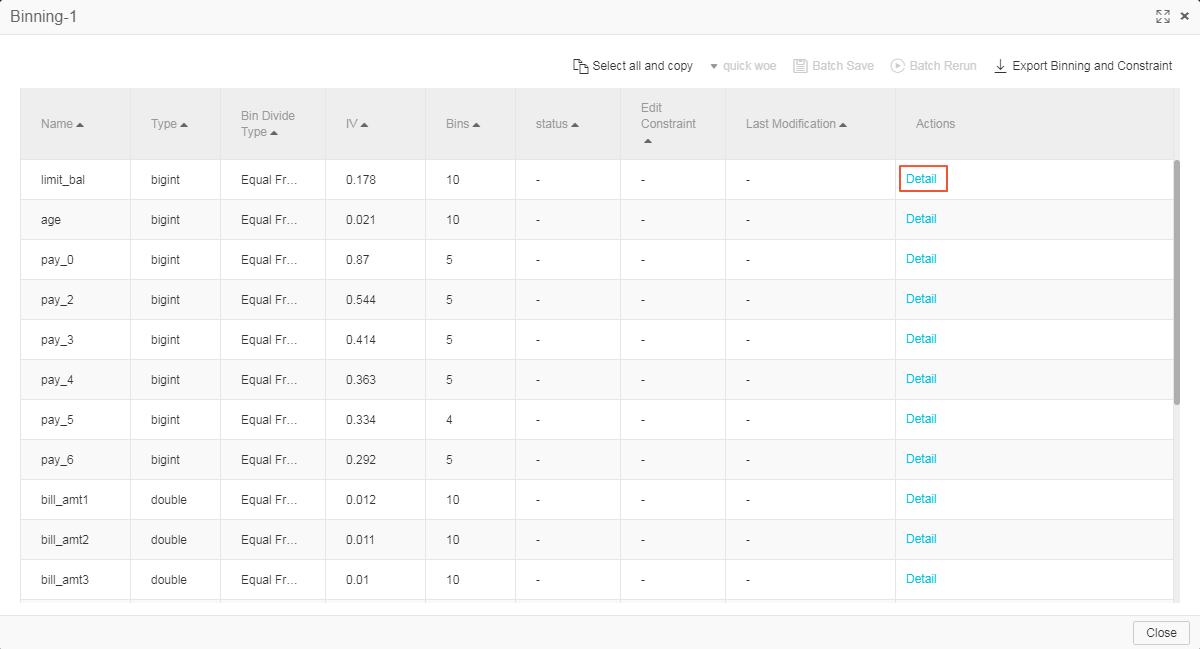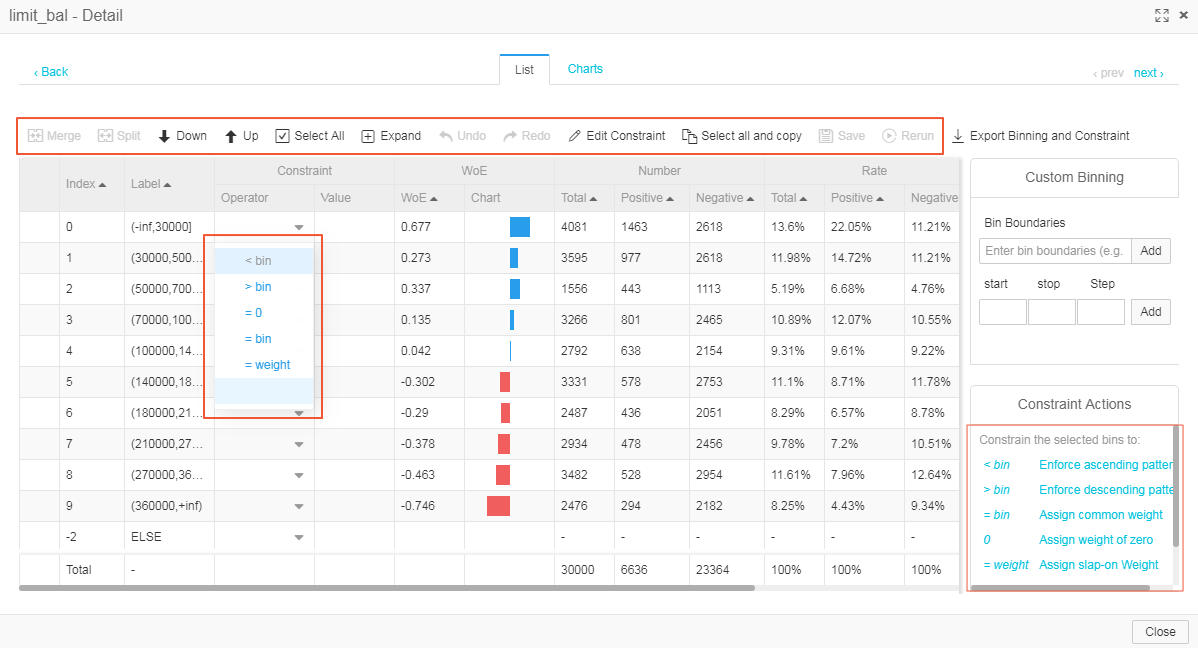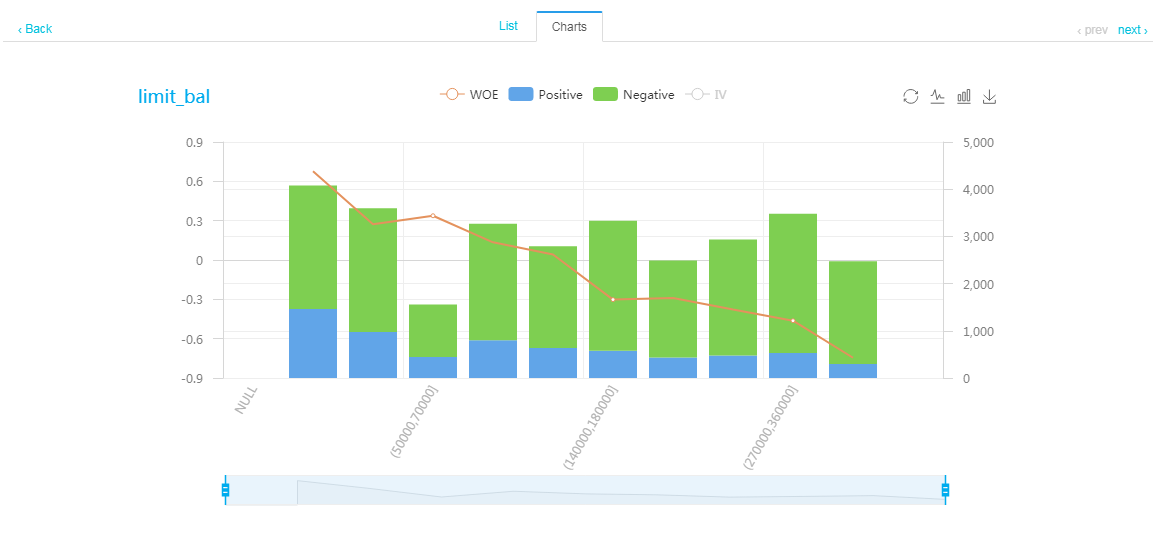Currently, the following JSON constraints are supported:

• “<”: The variable weights satisfy constraints in ascending order.
• “<”: The variable weights satisfy constraints in descending order.
• “=”: The variable weights are fixed values.
• “%”: The weights between variables meet a proportional relationship.
• “UP”: upper threshold of variable weight constraints.
• “LO”: lower threshold of variable weight constraints.

The JSON constraints are stored as a character string in a table. The table is single-row and single-column (character string), storing the following JSON character strings:

{    "name": "feature0",    "<": [        [0,1,2,3]    ],    ">": [        [4,5,6]    ],    "=": [        "3:0","4:0.25"    ],    "%": [        ["6:1.0","7:1.0"]    ]}

#### Built-in constraints

Each original variable has an implicit constraint, which does not need to be specified by the user. That is, the average score of a single variable’s population is 0. With this constraint, scaled_weight of the model intercept is the average score for the entire population.

#### Optimization algorithm

In the advanced options, you can select the optimization algorithm used in the training. Currently, the following optimization algorithms are supported:

• L-BFGS
• Newton’s Method
• Barrier Method
• SQP

L-BFGS is a first-order optimization algorithm that supports large-scale feature data. Newton’s method is a classic second-order algorithm that features fast convergence and high accuracy, but it is not suitable for large feature sizes because the second-order Hessian Matrix must be calculated. Both of the algorithms are unconstrained optimization algorithms. When these two optimization algorithms are selected, the constraints are automatically ignored.

Algorithm Sort Feature Others
L-BFGS first-order algorithm, unconstrained Supports large-scale feature data. When the optimization algorithms are selected, the constraints are automatically ignored.
Newton’s Method second-order algorithm, unconstrained Features fast convergence and high accuracy It is not suitable for large feature sizes because the second-order Hessian Matrix must be calculated. When the optimization algorithms are selected, the constraints are automatically ignored.
Barrier Method second-order algorithm, constrained The algorithms is similar with SQP in computational performance and accuracy. We recommend SQP by default. Equivalent to Newton’s method when no constraints are involved.
SQP second-order algorithm, unconstrained The algorithms is similar with Barrier Method in computational performance and accuracy. We recommend SQP by default. Equivalent to Newton’s method when no constraints are involved.

If you are unfamiliar with optimization algorithms, we recommend the default option “Automatic select”. An appropriate optimization algorithm is automatically selected based on the data size and constraints of user tasks.

#### Feature selection

The training module supports stepwise feature selection. Stepwise is a combination of forward selection and backward selection. After a new variable is selected to enter the model in each forward feature selection, backward selection needs to be performed on the variables in the model to remove the variables whose significance does not meet requirements. Because multiple objective functions and multiple feature transformation methods are supported, stepwise feature selection supports different selection criteria:

• Marginal contribution: Applies to all objective functions and feature engineering modes.
• Score test: Supports only WOE conversion or logistic regression selection without feature engineering.
• F test: Supports only WOE conversion or linear regression selection without feature engineering.

Marginal contribution

Concept

• Two models need to be trained. Model A does not include variable X, and model B includes variable X in addition to all variables of model A. The difference of objective functions in the final convergence of the two models is the marginal contribution between all variables of variable X in model B.

• In the scenario where feature engineering is a dummy transformation, the marginal contribution of X of the original variable is defined as the difference between objective functions of all the dummy variables when the two models respectively include and do not include the variable.

• Feature selection using marginal contribution supports all feature engineering modes.

Feature

• The advantage is that this method is flexible and is not limited to a certain model. The variables with an optimal objective function are directly selected to enter the model.

• The disadvantage is that marginal contribution is different from statistical significance. The threshold of statistical significance is typically 0.05, while no fixed threshold of marginal contribution is available for new users. The recommended default value is 10E-5.

Score test

Notice: The score test is applicable only to feature selection of logistic regression.

Forward selection:

1. Training a model with only the intercept firstly.

2. In each subsequent iteration, the Score Chi-Square for the variables that have not entered the model is calculated, the variable with the largest Score Chi-Square is selected to enter the model.

3. calculating the significance P Value based on the Score Chi-Square.

• If the P Value of the variable with the largest Score Chi-Square is greater than the maximum significance threshold (slentry) specified for entering the model, the variable is not included in the model and the selection stops.

• Else, go to forward selection.

Forward selection:

The backward selection is performed on variables that have been selected to enter the model.

In the backward selection, the Wald Chi-Square and the significance P Value are calculated for variables that have entered the model.

• If P Value is greater than the maximum significance threshold (slstay) specified for removal, the variable is removed from the model and the iteration selection starts.

• Else, including the variable in the model and start iteration selection.

F test

Notice: The F test is applicable only to feature selection of linear regression.

Forward selection

• Training variable with only the intercept firstly.

• In each subsequent iteration, F Values of the variables that have not entered the model are calculated.

F Value calculation is similar to edge contribution calculation. Two models are trained to calculate F Value of a variable.

• If F Value meets F distribution, the significance P Value can be calculated based on the probability density function of its F distribution.

• If P Value is greater than the maximum significance threshold (slentry) specified for entering the model, the variable is not included in the model and the selection stops.

• Else, go to forward selection.

Backward selection

Uses F Value to calculate the significance, and the process is similar to the score test.

Variables forcedly selected

Before feature selection, you can set variables that are forced into the model. Regardless of the significance, the selected variables directly enter the model and do not participate in the forward and backward feature selections.

The number of iterations and significance threshold are specified in CLI by using the -Dselected parameter in the JSON format:

{"max_step":2, "slentry": 0.0001, "slstay": 0.0001}

If this parameter is null or max_step is “0”, normal training is performed without feature selection.

We recommend that you use the scorecard on the PAI web. The following is a simple demonstration of comparison between STEPWISE feature selection and feature WOE transformation + logistic regression STEPWISE feature selection: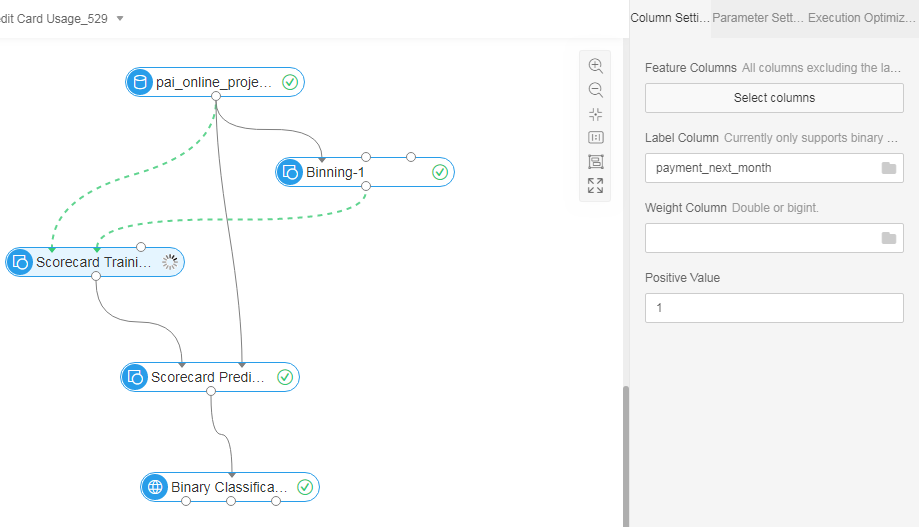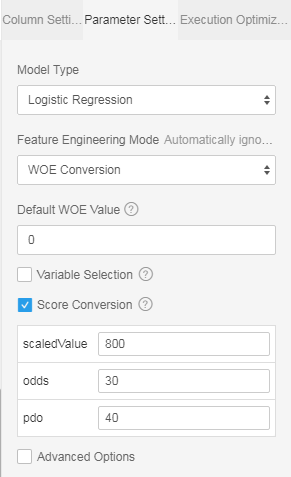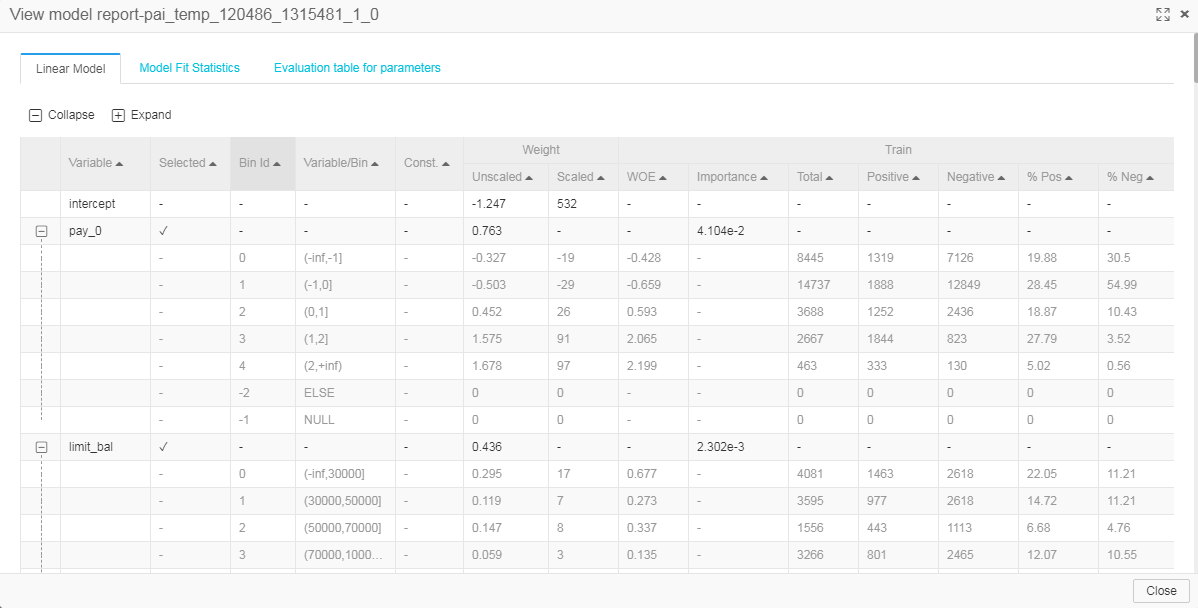#### Model report

The scorecard model output is a model report, which contains variable binning information, binning constraint information, basic statistical indicators such as WOE and marginal contribution, and scorecard model assessment reports displayed on the PAI web.

The related columns are described as follows:

Column Name Column Type Column Description
feaname string Feature name
binid bigint Bin ID
bin string Bin description, used to indicate the value range of the bin
constraint string Constraints added to the bin during training
weight double Bin variable weight after training or model variable weight in a non-scorecard model with no bin input specified
scaled_weight double Score value obtained after linear transformation of the bin variable weight when the score conversion information is specified in scorecard model training
Woe double Statistical indicator: WOE value of the bin in the training set
Contribution double Statistical indicator: marginal contribution value of the bin in the training set
total bigint Statistical indicator: total number of samples in the bin in the training set
positive bigint Statistical indicator: number of positive samples in the bin in the training set
negative bigint Statistical indicator: number of negative samples in the bin in the training set
percentage_pos double Statistical indicator: ratio of the number of positive samples to the total number of samples in the bin in the training set
percentage_neg double Statistical indicator: ratio of the number of negative samples to the total number of samples in the bin in the training set
test_woe double Statistical indicator: WOE value of the bin in the test set
test_contribution double Statistical indicator: marginal contribution value of the bin in the test set
test_total bigint Statistical indicator: total number of samples in the bin in the test set
test_positive bigint Statistical indicator: number of positive samples in the bin in the test set
test_negative bigint Statistical indicator: number of negative samples in the bin in the test set
test_percentage_pos double Statistical indicator: ratio of the number of positive samples to the total number of samples in the bin in the test set
test_percentage_neg double Statistical indicator: ratio of the number of negative samples to the total number of samples in the bin in the test set

#### Demonstration

The following is a simple demonstration of comparison between scorecard training and feature WOE conversion + logistic regression.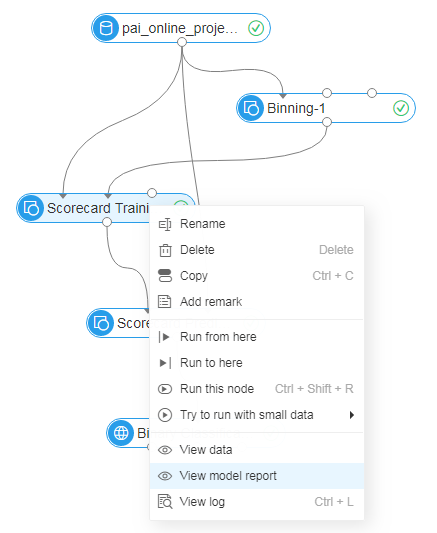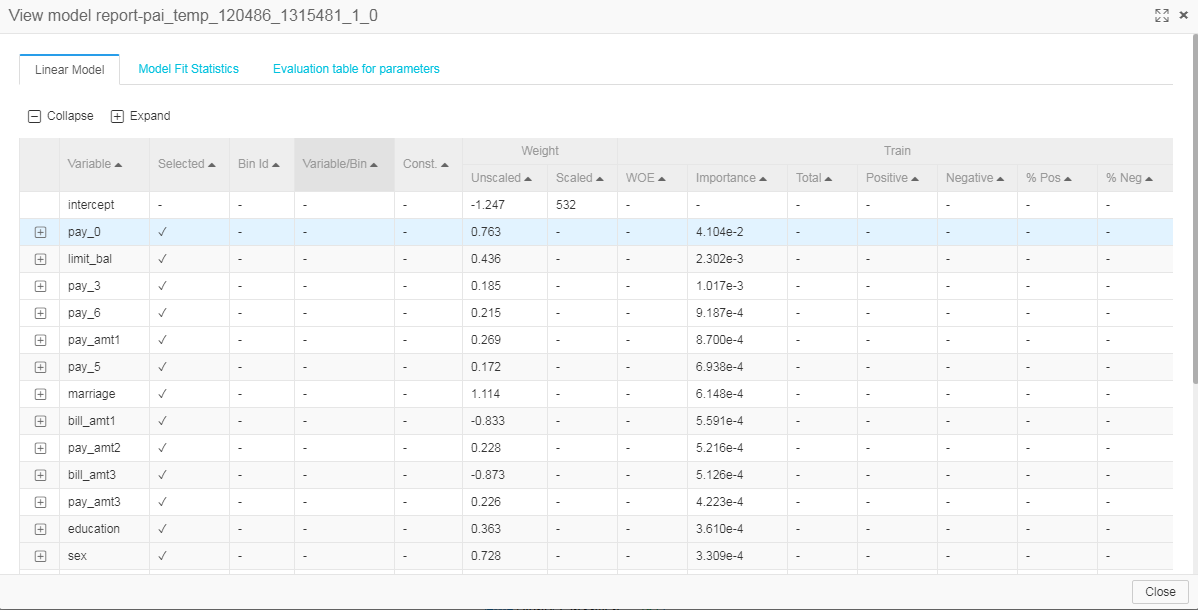When the test set is connected to the input training component, the output model report shows statistical indicators of the model in the test set, such as WOE and MC. The following is a simple training demonstration of a test set: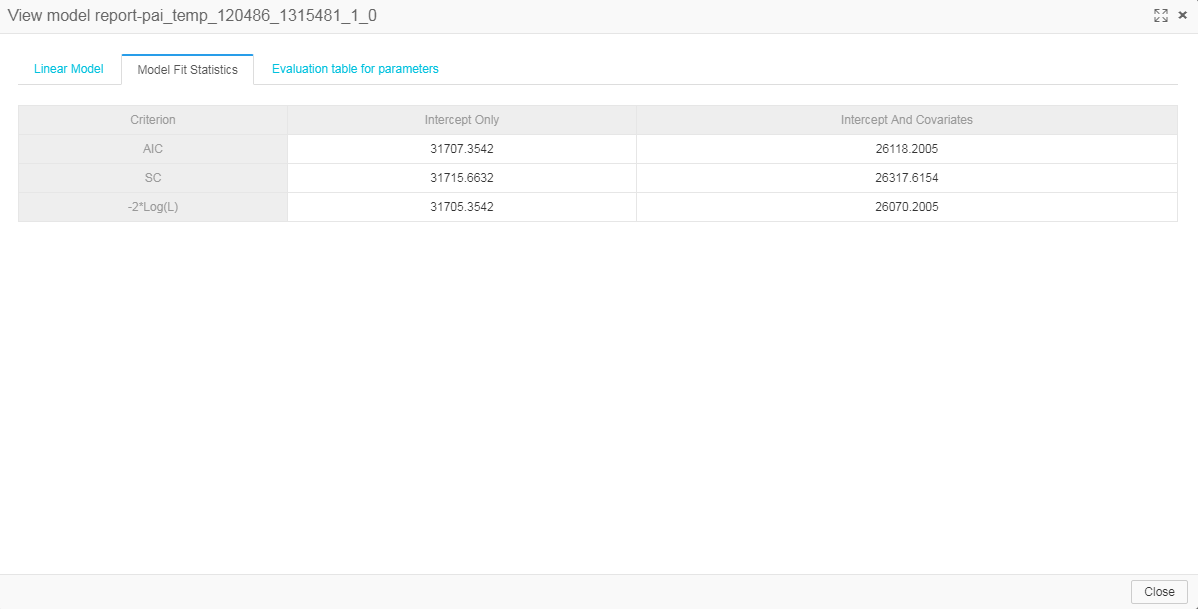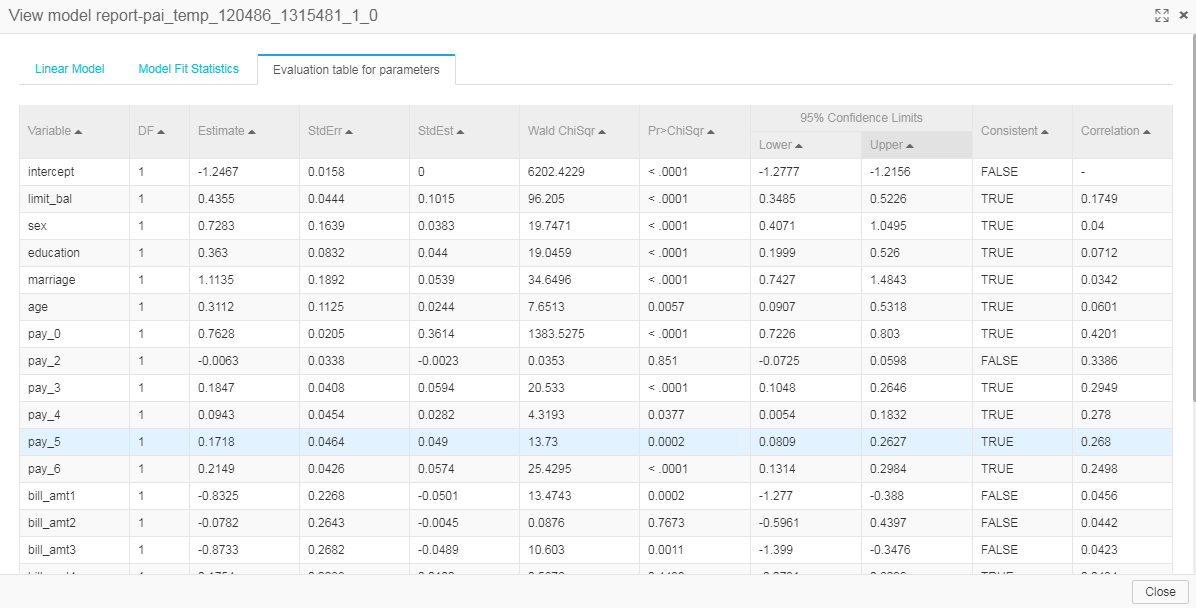#### PAI command

PAI -name=linear_model -project=algo_public    -DinputTableName=input_data_table    -DinputBinTableName=input_bin_table    -DinputConstraintTableName=input_constraint_table    -DoutputTableName=output_model_table    -DlabelColName=label    -DfeatureColNames=feaname1,feaname2    -Doptimization=barrier_method    -Dloss=logistic_regression    -Dlifecycle=8

#### Algorithm parameters

Parameter Description Option Default value
inputTableName (Required) Name of the input feature data table table name NA
inputTablePartitions (Optional) Partitions selected in the input feature data table partition name All partitions in the table by default
inputBinTableName (Optional) Input binning result table. If the table is specified, the original features are automatically discretized based on the binning rules of the table before training. table name NA
featureColNames (Optional) Feature columns selected in the input table column name All columns but the label column are selected by default.
labelColName (Required) Target column column name NA
outputTableName (Required) Name of the output model table table name NA
inputConstraintTableName (Optional) Input constraint in JSON format, stored in a cell of the table table name NA
optimization (Optional) optimization type Value options: lbfgs, newton, barrier_method, sqp, and auto. Currently, only sqp and barrier_method support constraints. auto is to automatically select an appropriate optimization algorithm based on user data and related parameters. We recommend that you select auto if you are unfamiliar with the preceding optimization algorithms. auto
loss (Optional) Loss type logistic_regression and least_square logistic_regression
iterations (Optional) Maximum number of optimization iterations positive integer Default value: 100
l1Weight (Optional) L1 regular parameter weight. Currently, only lbfgs supports l1weight. NA 0
l2Weight (Optional) L2 regular parameter weight NA 0
m (Optional) Historical length during lbfgs optimization, valid only for lbfgs NA 10
scale (Optional) Weight scale information on the scorecard NA Null
selected (Optional) Scorecard feature selection NA Null
convergenceTolerance (Optional) Convergence conditions NA 1e-6
positiveLabel (Optional) Class of the positive sample NA 1
lifecycle (Optional) Life cycle of the output table positive integer Unspecified by default
coreNum (Optional) Number of cores positive integer Automatically calculated by default
memSizePerCore (Optional) Size of memory positive integer Automatically calculated by default

## Scorecard prediction

• The scorecard prediction component predicts and scores raw data based on the output model produced by the training component.
• The supported training components include scorecard training, binary logistic regression (Financials), and linear regression (Financials).

#### Input parameters

The prediction component supports the following parameters:

• Feature column: Select the original feature columns used for prediction. By default, all columns are selected.

• Columns reserved in result table: Select the columns attached directly to the prediction result table, such as the common ID column and target column.

• Output variable score: Choose whether to output the score of each feature variable. The final total prediction score is the intercept score plus all the variable scores.

#### Output score table

The following is an example of an output score table: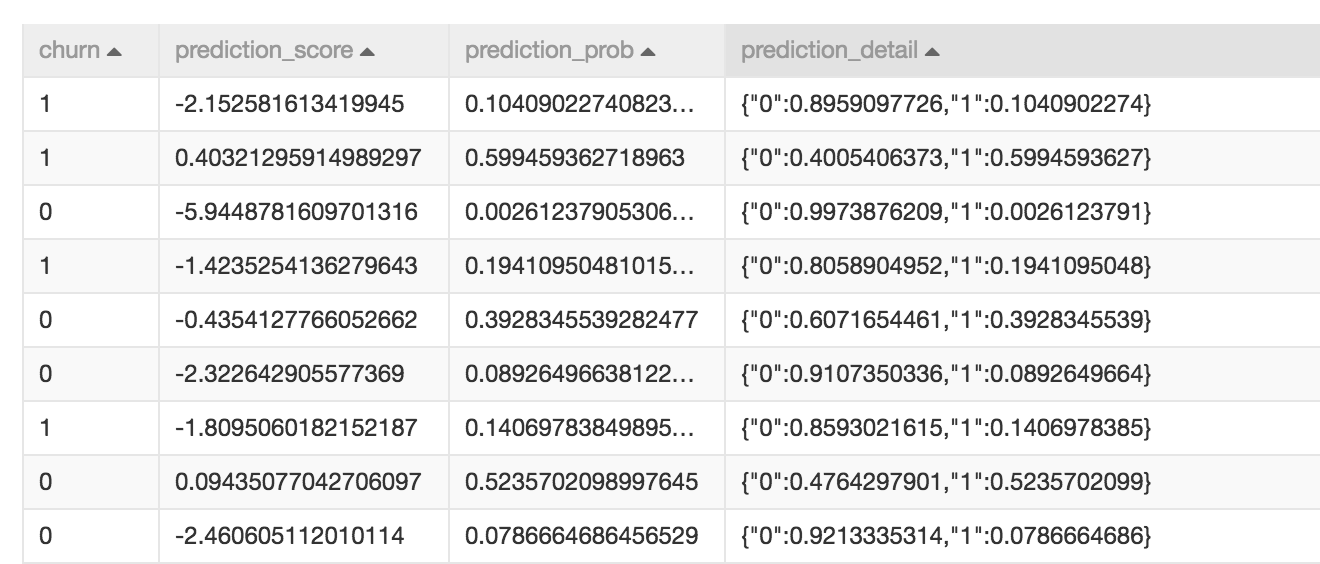The first column, churn, is added to the result table by the user as is and is irrelevant to the prediction result. The other three columns are prediction result columns, described as follows:

Column Name Column Type Column Description
prediction_score double Prediction score column, which lists the sum of feature values multiplied by model weights in the linear model. If score conversion is conducted in the scorecard model, converted scores are output.
prediction_prob double Positive probability value predicted in binary classification, obtained by sigmoid transformation of the raw score (without fraction conversion)
prediction_detail string Probability value of each class described in JSON format, where 0 represents a negative class and 1 represents a positive class. For example, {“0”:0.1813110520,”1”:0.8186889480}.

#### PAI command

PAI -name=lm_predict    -project=algo_public    -DinputFeatureTableName=input_data_table    -DinputModelTableName=input_model_table    -DmetaColNames=sample_key,label    -DfeatureColNames=fea1,fea2    -DoutputTableName=output_score_table

#### Algorithm parameters

Parameter Description Option Default value
inputFeatureTableName (Required) Name of the input feature data table table name NA
inputFeatureTablePartitions (Optional) Partitions selected in the input feature table partition name Entire table selected by default
inputModelTableName (Required) Name of the input model table table name NA
featureColNames (Optional) Feature columns selected in the input table column name Entire table selected by default
metaColNames (Optional) Data columns not converted. The selected column is output as is. column name No meta column by default, and you can specify label, sample_id, and other columns.
outputFeatureScore (Optional) Whether to include variable scores in the prediction result true/false false
outputTableName (Required) Name of the output prediction result table table name NA
lifecycle (Optional) Life cycle of the output table positive integer Unspecified by default
coreNum (Optional) Number of cores positive integer Automatically calculated by default
memSizePerCore (Optional) Size of memory positive integer Automatically calculated by default

## PSI

The population stability index (PSI) is an important indicator that measures the offset caused by sample changes. It is used to measure the sample stability, for example, whether a sample changes stably between two months.

Typically, if the PSI of a variable is less than 0.1, the variable does not have obvious changes. If the PSI is between 0.1 and 0.25, the variable changes greatly. If the PSI is greater than 0.25, the variable changes dramatically and requires special attention.

Drawings can be used to check the sample stability. Discretize the variables to be compared into N bins, calculate the number and proportion of samples in each bin, and draw a histogram based on the data, as shown in the following figure.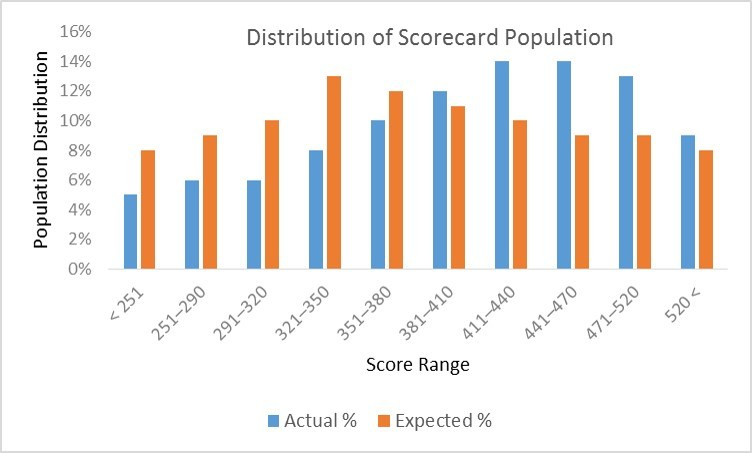This method helps you intuitively check whether a certain variable has drastic changes in the two samples. However, this method cannot quantify the changes or automatically monitor the sample stability. Therefore, the PSI is particularly important. The feature data must be divided into bins before the PSI can be used. Therefore, a binning component is required. The PSI calculation formula is as follows:#### Example

Connect the two sample data sets and binning component with PSI component. And set the parameter of the PSI component, choose Feature for PSI Calculation, as shown in the following figure.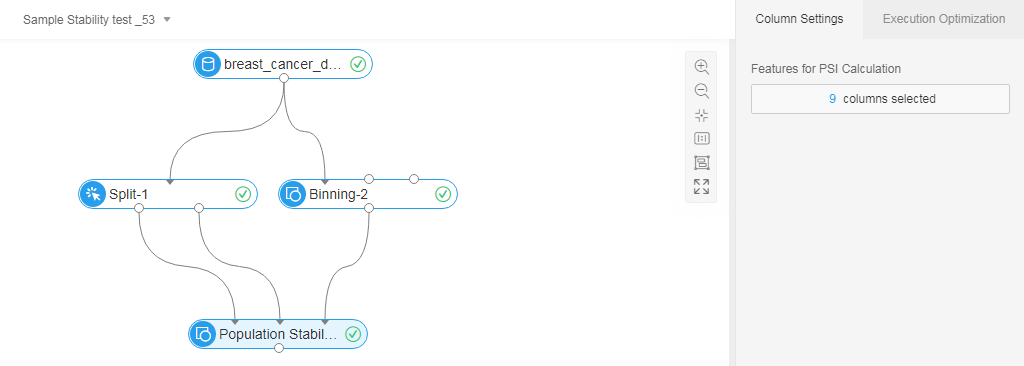#### Example of results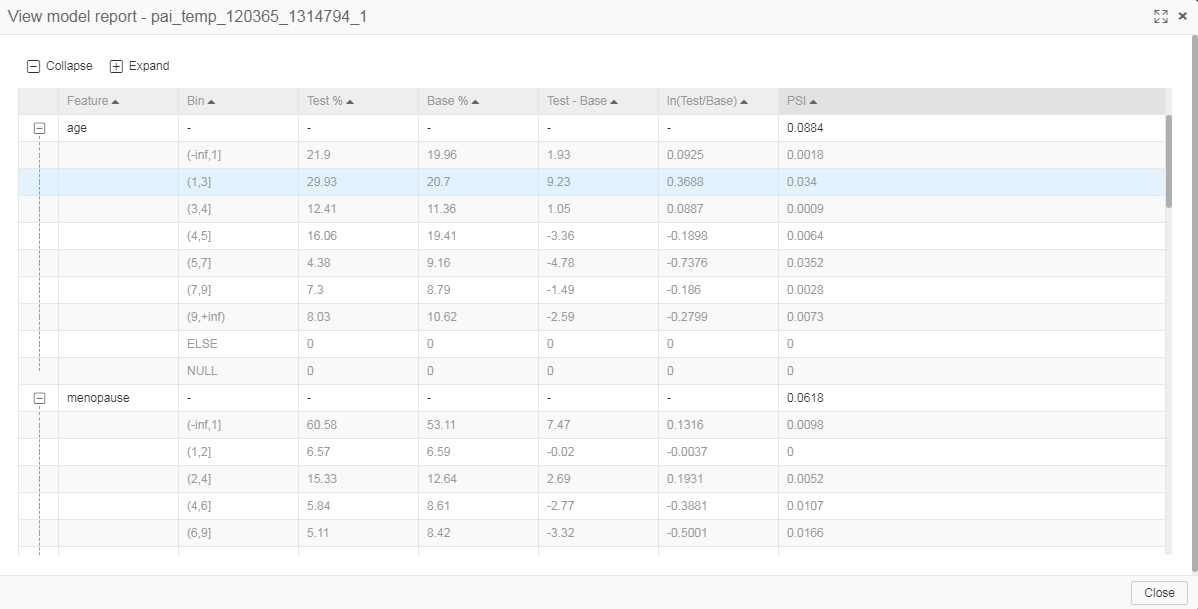#### PAI command

PAI -name psi-project algo_public-DinputBaseTableName=psi_base_table-DinputTestTableName=psi_test_table-DoutputTableName=psi_bin_table-DinputBinTableName=pai_index_table-DfeatureColNames=fea1,fea2,fea3-Dlifecycle=7

#### Algorithm parameters

Parameter Description Option Default value
inputBaseTableName (Required) Name of the input base table name. Calculate the offset of the test table relative to the base table. table name NA
inputBaseTablePartitions (Optional) Input base table partitions partition name Entire table selected by default
inputTestTableName (Required) Input test table name. Calculate the offset of the test table relative to the base table. table name NA
inputTestTablePartitions (Optional) Input test table partitions partition name Entire table selected by default
inputBinTableName (Required) Name of the input binning result table table name NA
featureColNames (Optional) Features of which the PSIs need to be calculated column name All features selected by default
outputTableName (Required) Output indicator table table name NA
lifecycle (Optional) Life cycle of the output table positive integer Unspecified by default
coreNum (Optional) Number of cores positive integer Automatically calculated by default
memSizePerCore (Optional) Size of memory positive integer Automatically calculated by default